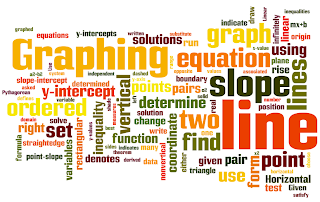Monday, November 14, 2011

Elementary Algebra (Ch.3) Graphing LinesElementary Algebra is an open textbook that is available to read for free on the flatworld knowledge website. Chapter 3 is an introduction to graphing linear equations. In this chapter, we learn how to graph lines in the rectangular coordinate plane and make connections between linear equations and lines. In addition, functions are introduced and linear inequalities are solved where the solutions are represented graphically and using interval notation.

3.8 Linear Inequalities (Two Variables)

In addition, you will find a complete review exercise section and a practice test.

3.9 Review Exercises and Sample Exam

Feel free to copy and paste the links found here and post them in your LMS.  Links to all of the chapters can be found by clicking the Elementary Algebra course button at the top of this page.

---

1 comment:

1.In mathematics a linear inequality is an inequality which involves a linear function. To solve Inequalities we must remember the symbols showing inequalities like the symbol < means less than and the symbol > means greater than and the symbol â‰¦ or ≤ less than or equal to etc.